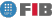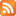Saltar al contingut Menu

# Theory of Computation (TC)

Credits Dept. Type Requirements
9.0 (7.2 ECTS) CS
• Compulsory for DIE
• Elective for DCSFW
ADA - Prerequisite for DIE , DCSYS
MATD - Prerequisite for DIE , DCSYS

## Instructors

 Person in charge: (-) Others: (-)

## General goals

The aim of this subject is to provide a theoretical structure allowing the analysis of processes in the basis on their difficulty. Studying the relationship between generativity (grammars) and solvability (automata), motivated by their use in compilers. Students should also acquire a theoretical knowledge of the limitations of these processes (undecidible problems). Upon finishing this subject, students should be familiar with the degrees of complexity intrinsic to regular and context-free languages. They will have a number of tools for describing, recognising, and characterising these languages.

## Specific goals

### Knowledges

1. Algorithm complexity and problem complexity.
2. Computation processes that only require a finite memory.

Finite automata.
3. Formal grammars. Analysis and compilation. Generativity.
4. Relationship between generative processes and recognition processes.
5. Hierarchical organisation of problems depending on their complexity.
6. The logical limits to computational capacity.
7. Undecidable problems.

### Abilities

1. Seeking a simpler computational model for each problem.
2. Tools for rejecting over-simplistic solutions for given problems.
3. Tools allowing calculation processes to be adequately described.

### Competences

1. Critical, logical-mathematical reasoning.
2. Ability to transform informal problems into formal ones and vice versa.
3. Ability to apply mathematical knowledge and logic in solving problems.
4. Ability to create and use models of reality.
5. Ability to analyze and summarise issues.

## Contents

Estimated time (hours):

 T P L Alt Ext. L Stu A. time Theory Problems Laboratory Other activities External Laboratory Study Additional time

1. Formal languages
T      P      L      Alt    Ext. L Stu    A. time Total
3,0 1,0 0 0 0 4,0 0 8,0

1. Introduction

2. Alphabets and words

3. Operations with words

4. Languages. Concatenation

5. Other operations with languages

6. Morphisms and substitutions

2. Non-contextual grammars
T      P      L      Alt    Ext. L Stu    A. time Total
4,0 6,0 0 0 0 10,0 0 20,0
1. Introduction

2. Definition

3. Derivation trees. Ambiguity

4. Grammar verification

5. Basic operations with grammars

6. The intersection of two CFLs cannot be a CFL (Context-Free Language).

3. Normalisation of grammars
T      P      L      Alt    Ext. L Stu    A. time Total
2,0 2,0 0 0 0 4,0 0 8,0

1. Introduction

2. Elimination of null checks

3. Elimination of bound checks

4. Elimination of useless symbols

5. Refined grammars

6. Chomsky"s normal form

4. Finite automata
T      P      L      Alt    Ext. L Stu    A. time Total
4,0 4,0 0 0 0 8,0 0 16,0

1. Introduction

2. Deterministic finite automata

3. Verification of finite automata

4. Indeterminate finite automata

5. Equivalent between NFAs and DFAs

6. Finite automata with lambda transitions

7. Basic operations with automata

8. Non-regular languages

5. Minimisation of finite automata
T      P      L      Alt    Ext. L Stu    A. time Total
2,0 2,0 0 0 0 4,0 0 8,0

1. DFA minimisation

2. Algorithm minimisation

3. Over the minimum DFA cut-off point

4. Automata equivalences

6. Regular expressions and regular grammars
T      P      L      Alt    Ext. L Stu    A. time Total
4,0 4,0 0 0 0 8,0 0 16,0

1. Introduction

2. Regular expressions

3. Linear equations between languages. Arden"s lemma

4. Systems of linear equations associated with an NFA

5. Regular grammars

6. Correspondence between regular grammars and finite automata

7. Morphisms and substitutions on regular languages

7. Iteration properties
T      P      L      Alt    Ext. L Stu    A. time Total
2,0 4,0 0 0 0 6,0 0 12,0

1. The pumping lemma of regular languages

2. Pumping lemmas in non-contextual languages

8. Stack automata
T      P      L      Alt    Ext. L Stu    A. time Total
4,0 2,0 0 0 0 6,0 0 12,0

1. Introduction

2. Deterministic stack automata

3. Non-deterministic stack automata

4. Equivalence between stack automata and non-contextual grammars

5. CFL and DCFL closure properties

9. Two--way automata and Chomsky hierarchy
T      P      L      Alt    Ext. L Stu    A. time Total
2,0 2,0 0 0 0 4,0 0 8,0

1. Introduction

2. Finite two-way automata

3. Two-way stack automata - 1.

4. Relationship between the studied languages

5. Chomsky hierarchy. Type 0 grammars

10. Turing Machines (TM)
T      P      L      Alt    Ext. L Stu    A. time Total
3,0 3,0 0 0 0 6,0 0 12,0
1. Introduction to calculability. Undecidable problems
2. Definition of a TM. Interpretation
3. Computation. Convergence and divergence
4. Language recognised and computed by a Turing Machine (TM)
5. TM - safe halt. Calculation time
6. Recursively enumerable languages and decidable languages
7. Computable functions
8. Extensions of the basic TM

11. Turing machines and algorithms
T      P      L      Alt    Ext. L Stu    A. time Total
2,0 2,0 0 0 0 4,0 0 8,0

1. Basic algorithm schemes

2. TM coding

3. Interpreters and simulators. The universal Turing Machine (TM)

4. The Church-Turing thesis.

12. Computability and decidability
T      P      L      Alt    Ext. L Stu    A. time Total
2,0 2,0 0 0 0 4,0 0 8,0

1. Projection Theorem

2. Closure properties of rp/decidable languages

3. Complementarity Theorem

4. Some r.p. languages The "K" programming language

5. Undecidable languages

13. Reducibility and Completeness
T      P      L      Alt    Ext. L Stu    A. time Total
2,0 5,0 0 0 0 7,0 0 14,0

1. Reduction between problems

2. Properties of reductions. Examples of reductions

3. Rice"s Theorem

4. Recursively enumerable complete sets

14. Some classic undecidable problems
T      P      L      Alt    Ext. L Stu    A. time Total
3,0 0 0 0 0 3,0 0 6,0

1. The Thue Problem (Word Problem)

2. The Post Office Problem

3. Undecidable problems in non-contextual languages

15. Constrained computation. Time and Space
T      P      L      Alt    Ext. L Stu    A. time Total
2,0 0 0 0 0 2,0 0 4,0

1. Calculation time

2. Calculation space

3. Classes of complexity

4. Properties

 Total per kind T P L Alt Ext. L Stu A. time Total 41,0 39,0 0 0 0 80,0 0 160,0 Avaluation additional hours 20,0 Total work hours for student 180,0

## Docent Methodolgy

The goal of the teaching and evaluation method is to increase students activity during the lectures, their own self-learning, and their learning in general.

The contents of the matter are not given along the class. These are given by means of videos posted on the web. The classes are used for solving student's doubts and doing continuous review.

## Evaluation Methodgy

The final evaluation of this course for a student is obtained from three grades, the final exam F (with an evaluation ranging between 0 and 10 points), three control exams C (with a total value ranging between 0 and 1 point), and the mark comming from the presentations of the student in the blackboard (between 0 and 2.5 points). The final mark of the subject is obtained by:

max(F,min(10,0.75F+C+P))

Note that the expression 0.75F+C+P may take a value of 11. This is why we get the minimum between it and 10. The maximum between F is get in order to do not punish the students making a good final exam but a bad continuous evaluation.

## Basic Bibliography

• Rafel Cases, Lluís Màrquez Llenguatges, gramàtiques i autòmats : curs bàsic, Edicions UPC, 2003.
• John E. Hopcroft, Rajeev Motwani, Jeffrey D. Ullman Introduction to automata theory, languages, and computation, Pearson Education International, 2003.
• Dean Kelley Automata and formal languages : an introduction, Prentice Hall, 1995.
• Maria Serna ... [et al.] Els Límits de la computació : indecidibilitat i NP-completesa, Edicions UPC, 2001.
• Michael Sipser Introduction to the theory of computation, Thomson Course Technology, 2006.

## Complementary Bibliography

• J. Glenn Brookshear Teoría de la computación : lenguajes formales, autómatas y complejidad, Addison-Wesley Iberoamericana, 1993.
• Joaquim Gabarró Vallès Informàtica clàssica : autòmats i gramàtiques, indecidibilitat, parallelisme massiu, Eumo, 1995.
• Jozef Gruska Foundations of computing, International Thomson Computer Press, 1997.
• Efim Kinber, Carl Smith Theory of computing : a gentle introduction, Prentice-Hall, 2001.
• Dexter C. Kozen. Automata and computability, Springer-Verlag,, 1997.
• Harry R. Lewis, Christos H. Papadimitriou Elements of the theory of computation, Prentice-Hall,, 1998.
• Dan A. Simovici, Richard L. Tenney Theory of formal languages with applications, World Scientific, 1999.
• Joan Vancells i Flotats Teoria d'autòmats i llenguatges formals I, Universitat Oberta de Catalunya, 2000.
• Derik Wood Theory of computation, Harper & Row, 1987.

(no available informacion)

## Previous capacities

Ability to express problems framed in terms of logical formulae in everyday language.
Ability to manipulate logical formulae.
Fundamental algebraic concepts: monoids, groups, closures, morphisms.
Basic principles of combinatorial algebra.
Ability to fully exploit the properties of Boolean algebra.
Understand basic data structures and the fundamental principles governing algorithms.
Ability to evaluate the temporal complexity of algorithms.© Barcelona school of informatics - Contact -RSS
This website uses cookies to offer you the best experience and service. If you continue browsing, it is understood that you accept our cookies policy.
Classic version Mobile version